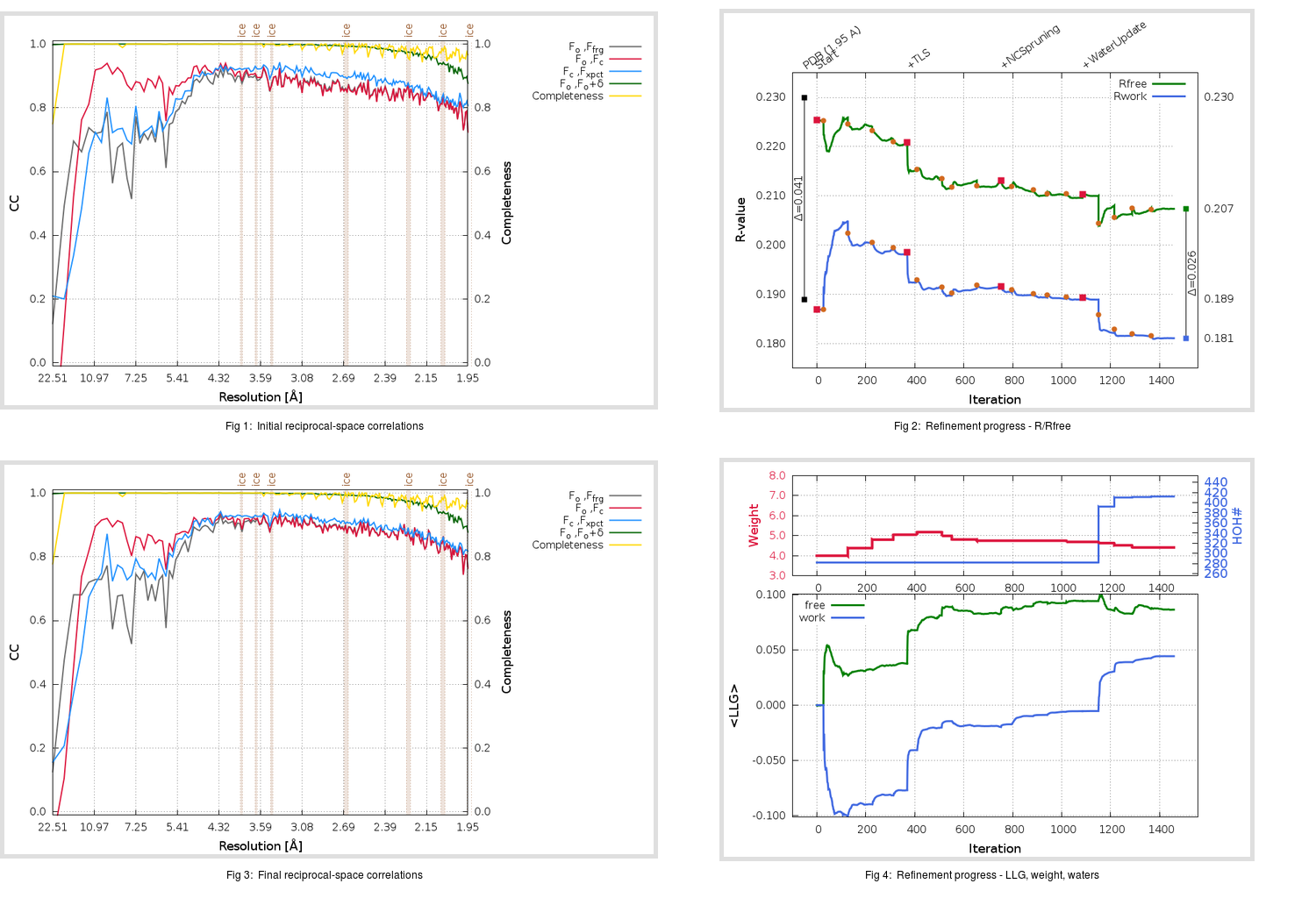Content:

## Deposited

` `
 Date deposited Date data collection Resolution R, Rfree 20111231 20091017 1.95 0.1880 0.2300

Molprobity (CCP4 7.0 version) summary:

```Ramachandran outliers =   0.33 %
favored =  96.86 %
Rotamer outliers      =   1.71 %
C-beta deviations     =     3
Clashscore            =   6.44
RMS(bonds)            =   0.0074
RMS(angles)           =   1.14
MolProbity score      =   1.72
Resolution            =   1.95
R-work                =   0.1880
R-free                =   0.2300
```

```Number of waters      =   282

<B> (all atoms) =   29.72 ( sd =   11.47 ) for       5061 non-hydrogen atoms
<B>   (protein) =   29.47 ( sd =   11.64 ) for       4743 non-hydrogen atoms
<B>     (water) =   33.39 ( sd =    7.87 ) for        282 non-hydrogen atoms
<B>    (others) =   34.23 ( sd =    5.46 ) for         36 non-hydrogen atoms

B min/max       (all non-hydrogen atoms) =   11.51 /   88.54
B min/max   (protein non-hydrogen atoms) =   11.51 /   88.54
B min/max     (water non-hydrogen atoms) =   15.43 /   54.99
B min/max     (other non-hydrogen atoms) =   25.49 /   48.76
```

## BUSTER (re-)refinement

` `

Molprobity (CCP4 7.0 version) summary:

```Ramachandran outliers =   0.00 %
favored =  98.18 %
Rotamer outliers      =   2.28 %
C-beta deviations     =     1
Clashscore            =   4.01
RMS(bonds)            =   0.0117
RMS(angles)           =   1.53
MolProbity score      =   1.46
Resolution            =   1.95
R-work                =   0.1811
R-free                =   0.2074
```

```Number of waters      =   412

<B> (all atoms) =   32.52 ( sd =   12.22 ) for       5191 non-hydrogen atoms
<B>   (protein) =   31.61 ( sd =   11.92 ) for       4743 non-hydrogen atoms
<B>     (water) =   42.00 ( sd =   11.50 ) for        412 non-hydrogen atoms
<B>    (others) =   43.21 ( sd =    7.89 ) for         36 non-hydrogen atoms

B min/max       (all non-hydrogen atoms) =   13.51 /   89.14
B min/max   (protein non-hydrogen atoms) =   13.51 /   89.14
B min/max     (water non-hydrogen atoms) =   17.11 /   76.39
B min/max     (other non-hydrogen atoms) =   34.22 /   63.54
```

Refinement progression:Results:

` `
 File Remark 3VB7_aB_refine.01_04_refine.pdb.gz exact refinement commands are in header 3VB7_aB_refine.01_04_refine.mtz.gz including original deposited data and several re-refinement map coefficients 3VB7_aB_refine.01_04_BUSTER_model.cif.gz including any non-standard compound restraints 3VB7_aB_refine.01_04_BUSTER_refln.cif.gz